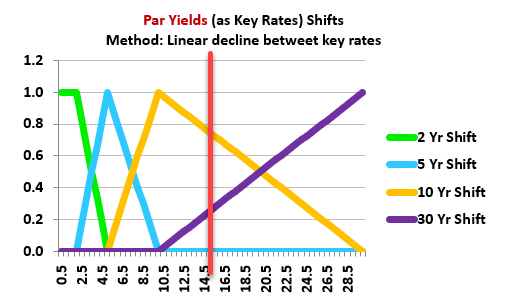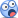# Key rates defined in terms of par yields infer the hedge position

#### rohinjain

##### Member
Hi David

On page 170 of VRM, GARP says that when key rates are defined in terms of par yields, one can immediately calculate the position necessary to hedge a portfolio once the exposure of the portfolio to the key rates are calculated.

I think I am struggling to see why all the changes in the par yields should add up to the yield-based DV01 (as the book mentions on p170). I understand that making a 1bp change in all the spot rates is equivalent to making a 1bp change in all the yields, but I am not sure how this links to par yields, yield-based DV01 and computing the necessary hedge positions.

Rohin

#### David Harper CFA FRM

##### David Harper CFA FRM
Staff member
Subscriber
Hi @rohinjain If par yields are used, the sum of KR01's will not exactly equal the DV01, so it should probably say "The three changes add up approximately to a yield-based DV01." But this is a (very) subtle point due to the nature of a par yield. The more common misunderstanding here is how the decision rule of linear interpolation implies that the sum of KR01s (whether the key rates are spot rate, par yields, or forward rates) should approximate the DV01. See below which mimics Tuckman's selection of four par yield key rates. My red vertical line is drawn at 15.0 years. Like almost all maturities (except 2.0, 5.0, 10.0 and 30.0 years), the technique recognizes that 15.0 years is a neighbor to both they 10.0 and 30.0 year key rates. In regard to the 10-year par yield key rate, it will get a shock of +0.0075% because it is 1/4 of the way from 10.0 to 30 years; in regard to the 30-year key rate it will get a shock of +0.0025% for the same reason. Each KR01(10) and KR01(30) will illustrate its own impact, but when we add up all four every maturity on the term structure (as seen by any vertical line) receives a total of +0.010% (one basis point) shock. In this way, it must approximate the parallel shift of DV01. As my learning XLS illustrates, the shocked par yields must be translated into the spot rates (aka, discount factors) in order to re-price the bond.Unless you want to examine the learning XLS, I recommend relying on the intuitive approximation (i.e., each maturity by virtue of being a neighbor gets exactly plus one basis point shock regardless of the key rate selected).

The advantage of par yields as key rates is a different aspect (as much discussed over the years); confusion here is almost always due to an inexpert understanding of par yields. Here is my recent video on understanding par yields https://forum.bionicturtle.com/threads/t3-13-par-yields-are-swap-rates.22426/ The advantage of par yields is that it is convenient to find the hedging portfolio if the hedging portfolio can be par-priced bonds with matrurities equal to the key rate maturities (e.g., 2-year par bond, 5-year par bonds ...). Here is the key (pun intended!) section where Tuckman explain this:
"If the maturity of a coupon bond were exactly equal to the term of a key rate and if the price of that bond were exactly par, then that bond’s yield would be identical to that key rate. By definition, then, that bond’s key-rate ’01 with respect to that key rate would equal its yield-based DV01 while its key-rate ’01 with respect to all other key rates would be zero." -- Tuckman, Bruce. Fixed Income Securities: Tools for Today's Markets (Wiley Finance) (p. 160). Wiley. Kindle Edition.
... this is a security-level point: these illustrated par yield key rates (2, 5, 10 and 30 years) signify an intention to hedge with 2-, 5-, 10, and 30-year par (priced but coupon paying) bonds. Conveniently, each of these par bonds has a KR01 equal to its DV01. This makes solving for the hedging portfolio (that includes four bonds) easier. But this hedging motivative comes at the cost of complicating an understanding of the key rate shift (because the par yield shifts must still be translated in a discount function). It's a different matter (i.e., more global and true regardless of which type of key rate) that, when you add up the KR01s, they will approximate the single-factor DV01 implied by a single parallel shift. I hope that's helpful,

Last edited:

#### rohinjain

##### Member
Top-notch explanation @David Harper CFA FRM ! I think I got it now... it took a while